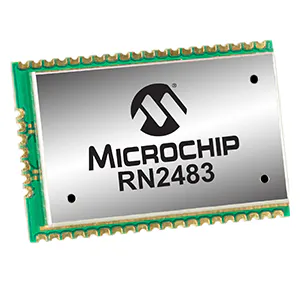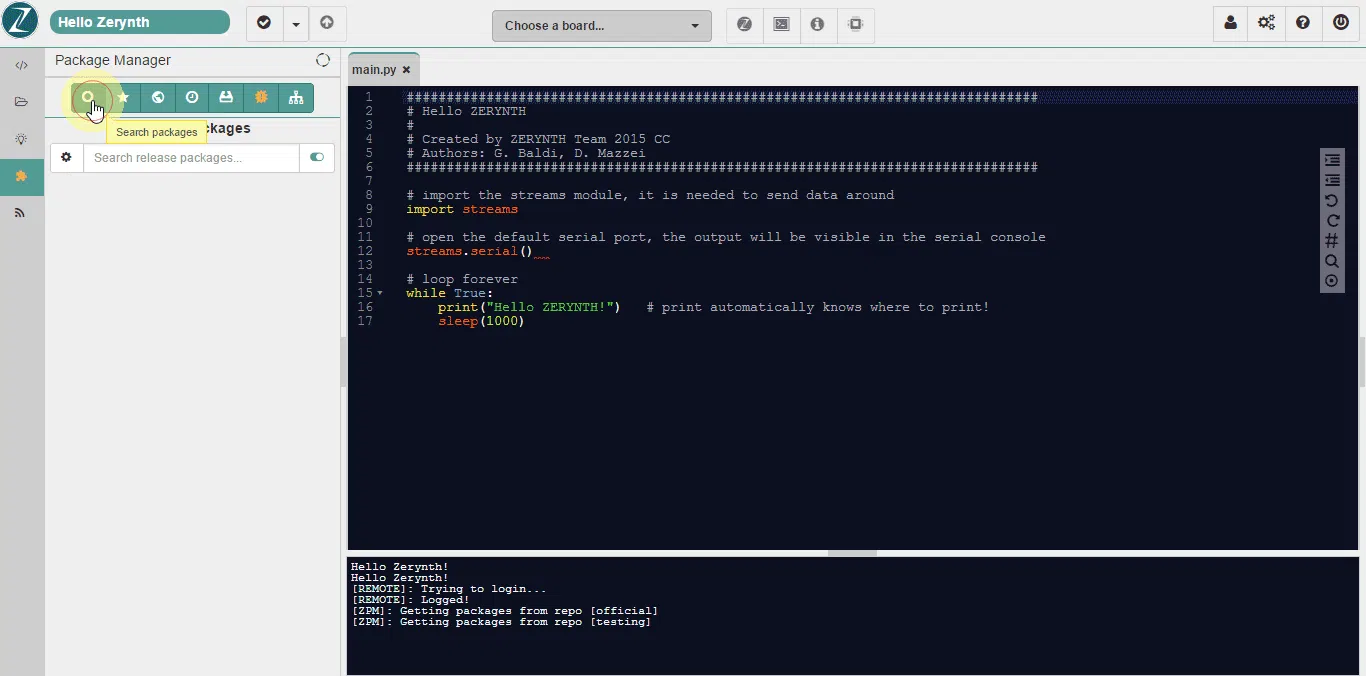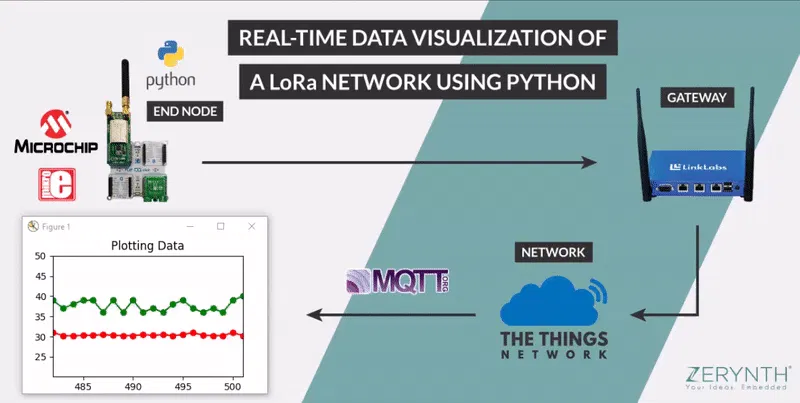# Real-Time Data Plotting of LoRa Nodes Using Python

In this article, we'll see how to get and visualize sensor data of LoRa nodes using Zerynth, The Things Network, and Matplotlib.

IntermediateFull instructions provided2 hours3,550

## Things used in this project

### Hardware components

 MikroElektronika Flip&Click
×1
 MikroElektronika LoRa Click
×1
 MikroElektronika Temp&Hum Click
×1Microchip Technology RN2483
×1

### Software apps and online servicesZerynth StudioThe Things Network

## Schematics

### Zerynth and TTN - LoRa## Code

### LoraWAN sensor data Logging

Python
```# LoraWAN sensor data Logging

import streams
from microchip.rn2483 import rn2483
from stm.hts221 import hts221

streams.serial()

try:
rst = D16 # reset pin
# insert otaa credentials!
appeui = "YOUAPPEUI"
appkey =  "YOUAPPKEY"
print("joining...")

if not rn2483.init(SERIAL1, appeui, appkey, rst): # LoRa Click on slot A
print("denied :(")
raise Exception

print("sending first message, res:")
print(rn2483.tx_uncnf('TTN'))

temp_hum = hts221.HTS221( I2C1,D31 ) # Temp Hum Click on slot C

while True:
temp, hum = temp_hum.get_temp_humidity()
print('temp: ', temp, 'hum: ', hum)
data = bytearray(4)
data[0:2] = bytearray([ int(temp) + 127, int((temp - int(temp)) * 100) ])
data[2:4] = bytearray([ int(hum) + 127, int((hum - int(hum)) * 100) ])
r = rn2483.tx_uncnf(data) # send data to TTN
sleep(5000)

except Exception as e:
print(e)
```

### get_plot_data_TTN.py

Python
```# Get and plot data from TTN Console using Python

import paho.mqtt.client as mqtt
import json
import base64

APPEUI = 'YOURAPPEUI'
APPID  = 'YOUAPPID'

import matplotlib.pyplot as plt
#import DataPlot and RealtimePlot from the file plot_data.py
from plot_data import DataPlot, RealtimePlot

fig, axes = plt.subplots()
plt.title('Data from TTN console')

data = DataPlot()
dataPlotting= RealtimePlot(axes)

count=0

def bytes_to_decimal(i,d):
xx = i - 127
dec = (-d if xx < 0 else d)/100
return xx + dec

def on_connect(client, userdata, flags, rc):
client.subscribe('+/devices/+/up'.format(APPEUI))

def on_message(client, userdata, msg):
dev_eui = j_msg['hardware_serial']

tmp = bytes_to_decimal(*tmp_hum[0:2])
hum = bytes_to_decimal(*tmp_hum[2:4])

# print data
print('---')
print('tmp:', tmp, ' hum:', hum)
print('dev eui: ', dev_eui)

# plot data
global count
count+=1
dataPlotting.plot(data)
plt.pause(0.001)

# set paho.mqtt callback
ttn_client = mqtt.Client()
ttn_client.on_connect = on_connect
ttn_client.on_message = on_message
ttn_client.connect("eu.thethings.network", 1883, 60) #MQTT port over TLS

try:
ttn_client.loop_forever()
except KeyboardInterrupt:
print('disconnect')
ttn_client.disconnect()
```

### plot_data.py

Python
```import time
import math
from collections import deque , defaultdict

import matplotlib.animation as animation
from matplotlib import pyplot as plt

from random import randint

from statistics import *

class DataPlot:
def __init__(self, max_entries = 20):
self.axis_x = deque(maxlen=max_entries)
self.axis_y = deque(maxlen=max_entries)
self.axis_y2 = deque(maxlen=max_entries)

self.max_entries = max_entries

self.buf1=deque(maxlen=5)
self.buf2=deque(maxlen=5)

self.axis_x.append(x)
self.axis_y.append(y)
self.axis_y2.append(y2)

class RealtimePlot:
def __init__(self, axes):

self.axes = axes

self.lineplot, = axes.plot([], [], "ro-")
self.lineplot2, = axes.plot([], [], "go-")

def plot(self, dataPlot):
self.lineplot.set_data(dataPlot.axis_x, dataPlot.axis_y)
self.lineplot2.set_data(dataPlot.axis_x, dataPlot.axis_y2)

self.axes.set_xlim(min(dataPlot.axis_x), max(dataPlot.axis_x))
ymin = min([min(dataPlot.axis_y), min(dataPlot.axis_y2)])-10
ymax = max([max(dataPlot.axis_y), max(dataPlot.axis_y2)])+10
self.axes.set_ylim(ymin,ymax)
self.axes.relim();

def main():
fig, axes = plt.subplots()
plt.title('Plotting Data')

data = DataPlot();
dataPlotting= RealtimePlot(axes)

try:
count=0
while True:
count+=1
data.add(count, 30 + 1/randint(1,5) , 35 + randint(1,5))
dataPlotting.plot(data)

plt.pause(0.001)
except KeyboardInterrupt:
plt.close()
ser.close()
exit()

if __name__ == "__main__": main()
```

## Credits

### Luigi Francesco Cerfeda

6 projects • 83 followers
Yet another Tony Stark wannabe